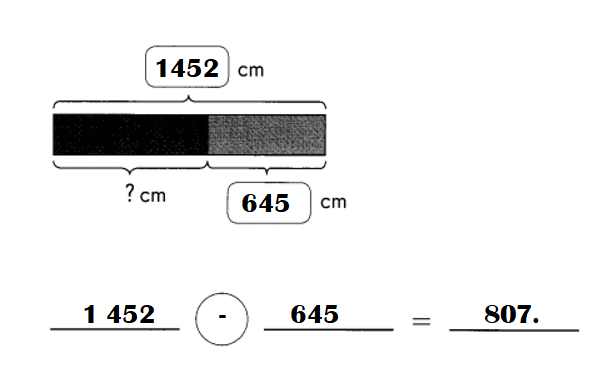# Math in Focus Grade 3 Chapter 5 Practice 1 Answer Key Real-World Problems: Addition and Subtraction

Go through the Math in Focus Grade 3 Workbook Answer Key Chapter 5 Practice 1 Real-World Problems: Addition and Subtraction to finish your assignments.

## Math in Focus Grade 3 Chapter 5 Practice 1 Answer Key Real-World Problems: Addition and Subtraction

Example
A biking team raises $4,250 for charity. A running team raises$825 more than the biking team.

a. How much money does the running team raise?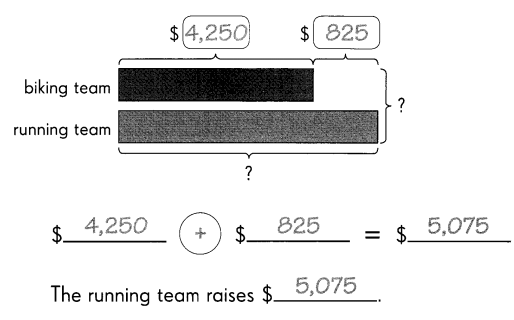b. How much money do both teams raise in all?
$4,250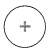$ 5,075 = $9,325 Both teams raise$9,325 in all.

Question 1.
The third graders collect 487 cans of food for a food drive. The second graders collect 175 fewer cans than the third graders.
a. How many cans do the second graders collect?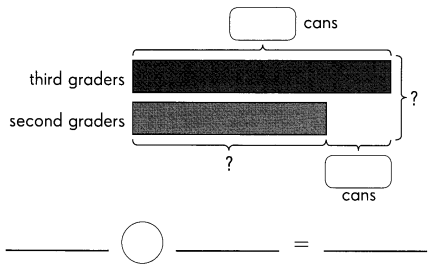The second graders collect __________ cans.
Number of cans of food for a food drive the second graders collect = 312.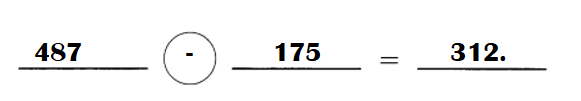Explanation:
Number of cans of food for a food drive the third graders collect = 487.
=> Number of cans of food for a food drive the second graders collect = Number of cans of food for a food drive the third graders collect – 175
= 487 – 175
= 312.

b. How many cans do both grades collect in all?
___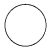___ = ____
Both grades collect ___ cans in all.
Total number of cans of food for a food drive the second and third graders collect = 799.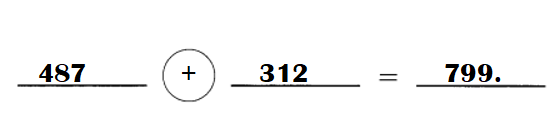Explanation:
Number of cans of food for a food drive the third graders collect = 487.
Number of cans of food for a food drive the second graders collect = 312.
Total number of cans of food for a food drive the second and third graders collect = Number of cans of food for a food drive the third graders collect + Number of cans of food for a food drive the second graders collect
= 487 + 312
= 799.

Question 2.
A bookstore has 4,320 books and magazines. It has 2,169 books. The rest are magazines.
a. How many magazines does the bookstore have?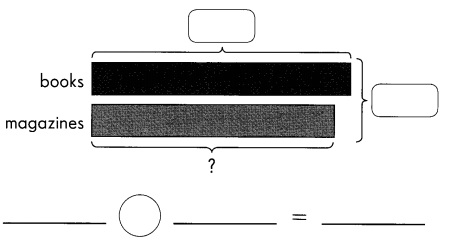The bookstore has ___ magazines.
Number of magazines the bookstore has = 2151.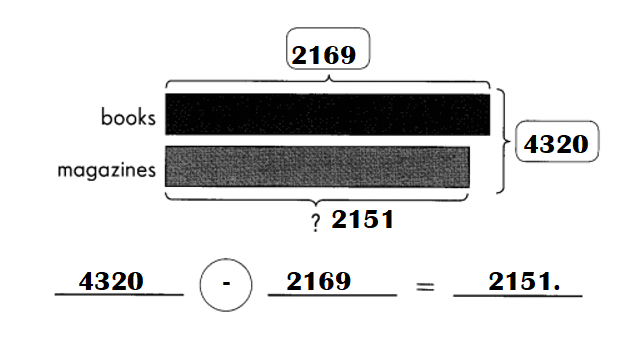Explanation:
Number of books and magazines a bookstore has = 4,320.
Number of books it has = 2,169.
Number of magazines the bookstore has = Number of books and magazines a bookstore has – Number of books it has
= 4320 – 2169
= 2151.

b. There are 1,493 fashion magazines. The rest are sports magazines.
How many sports magazines does the bookstore have?The bookstore has ___ sports magazines.
The bookstore has 658 sports magazines.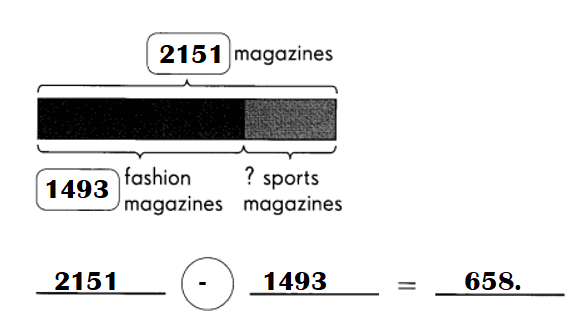Explanation:
Number of magazines the bookstore has = 2151.
Number of fashion magazines = 1493.
Number of sports magazines the bookstore has = Number of magazines the bookstore has –  Number of fashion magazines
= 2151 – 1493
= 658.

Question 3.
Ray’s rope is 1 452 centimeters long. Hannah’s rope is 379 centimeters longer than Ray’s rope.

a. How long is Hannah’s rope?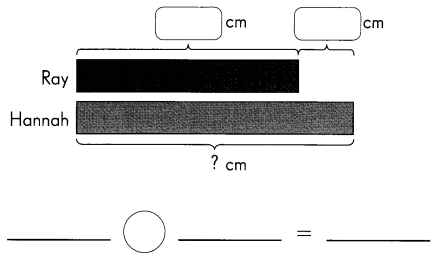Hannah’s rope is ____ centimeters long.
Hannah’s rope is 1831.centimeters long.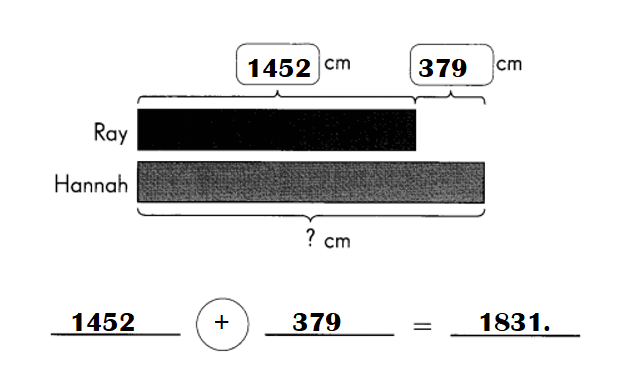Explanation:
Number of centimeters long Ray’s rope = 1 452.
Hannah’s rope is 379 centimeters longer than Ray’s rope.
=> Number of centimeters long Hannah’s rope = Number of centimeters long Ray’s rope + 379
= 1452 + 379
= 1831.

b. Ray uses 645 centimeters of his rope. How long is his remaining rope?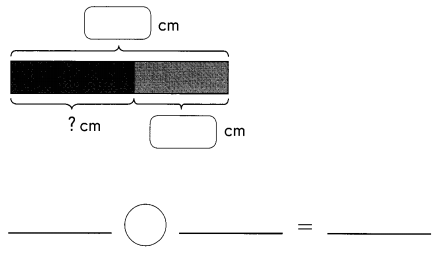Ray’s remaining rope is ___ centimeters long.